# 201871010129-郑文潇 实验三 结对项目—《D{0-1}KP 实例数据集算法实验平台》项目报告

#### 任务一

##### 代码设计规范

1.函数 现代程序设计语言中的绝大部分功能,都在程序的函数中实现。
2.goto 函数最好有单一的出口，为了达到这一目的，可以使用goto。只要有助于程序逻辑的清晰体现，什么方法都可以使用。
3.错误处理 当程序的主要功能实现后，给代码加一些错误处理。
4.参数处理 在Debug版本中，所有的参数都要验证其正确性。在正式版本中，对从外部(用户或别的模块)传递过来的参数，要验证其正确性。
5.断言 当你觉得某事肯定如何时，就可以用断言。

##### 代码复审
1. 找出代码的错误
2. 发现逻辑错误
3. 发现算法错误
4. 发现潜在的错误和回归性错误
5. 发现可能需要改进的地方
6. 开发人员，传授经验，在交流中不断提升能力。

#### 任务二

1.已对我的结对放谢金鑫实验二进行了中肯的评价

2.克隆结对方项目源码到本地机器，阅读并测试运行代码，参照《现代软件工程—构建之法》4.4.3节核查表复审同伴项目代码并记录。

#### 任务三

##### 2.核心代码：

（1）初始界面

</head>

<body>

<font size="5">

<a href="sandain.html" title="读者你好，单击这里可以发电子邮件。">散点图</a><br />

<a href="dongtai.html" title="读者你好，欢迎进入FTP服务器。">动态规划</a><br />

<a href="huisu.html" title="读者你好，欢迎进入Telnet服务器。">回溯法</a><br />

<a href="yichuan.html" title="读者你好，欢迎进入Telnet服务器。">遗传算法</a>
</font>

</body>

</html>


（2）动态规划

def dp(num, maxWeight):
l = []
profitArr = []
profitArr = profit[num]
weightArr = []
weightArr = weight[num]
for i in range(maxWeight + 1):
l.append(0)
for i in range(len(profit[num])):
for j in range(maxWeight, -1, -1):
for k in range(3):
if j >= weightArr[i][k]:
l[j] = max(l[j], l[j - weightArr[i][k]] + profitArr[i][k])
print(l[maxWeight])



（3）回溯法

def huisu(num, maxWeight, x, y, totalP, totalW):  # 访问一个节点   x,y 计算当前价值
if y != 3:
totalP = totalP + profit[num][x][y]
totalW = totalW + weight[num][x][y]
if x == len(profit[num]) - 1:
# 总价值和总重量
if totalW > maxWeight:
# print(totalP)
pathList.append(totalP)
return 0
else:
endMax.append(totalP)
pathList.append(totalP)
return 0
else:
for i in range(4):
huisu(num, maxWeight, x + 1, i, totalP, totalW)
return 0



（4）遗传算法

# coding=utf-8
import random

#背包问题
# 物品 重量 价格
X = {
1: [10, 15],
2: [15, 25],
3: [20, 35],
4: [25, 45],
5: [30, 55],
6: [35, 70]}

#终止界限
FINISHED_LIMIT = 5

#重量界限
WEIGHT_LIMIT = 80

#染色体长度
CHROMOSOME_SIZE = 6

#遴选次数
SELECT_NUMBER = 4

max_last = 0
diff_last = 10000

#判断退出
def is_finished(fitnesses):
global max_last
global diff_last
max_current = 0
for v in fitnesses:
if v > max_current:
max_current = v
print 'max_current:',max_current  # 得到当前最大的价值
diff = max_current - max_last # 价值差，也就是适应度的改变的大小
# 这里判断连续两代的改变量如果都小于5，则停止迭代
if diff < FINISHED_LIMIT and diff_last < FINISHED_LIMIT:
return True
else:
diff_last = diff
max_last = max_current
return False

#初始染色体样态
def init():
chromosome_state1 = '100100'
chromosome_state2 = '101010'
chromosome_state3 = '010101'
chromosome_state4 = '101011'
chromosome_states = [chromosome_state1,
chromosome_state2,
chromosome_state3,
chromosome_state4]
return chromosome_states

#计算适应度
def fitness(chromosome_states):
fitnesses = []
for chromosome_state in chromosome_states: # 遍历所有的染色体
value_sum = 0  # 物品重量
weight_sum = 0 # 物品价值
# 将一个可遍历的数据对象组合为一个索引序列，同时列出数据和数据下标
for i, v in enumerate(chromosome_state):
# 对染色体中的1，即存在的物品体重和价格求和
if int(v) == 1:
weight_sum += X[i + 1]
value_sum += X[i + 1]
fitnesses.append([value_sum, weight_sum])
return fitnesses

#筛选
def filter(chromosome_states, fitnesses):
#重量大于80的被淘汰
index = len(fitnesses) - 1
while index >= 0:
index -= 1
if fitnesses[index] > WEIGHT_LIMIT:
chromosome_states.pop(index) # 弹出不符合条件的染色体
fitnesses.pop(index)  # 弹出不符合条件的适应度
#print chromosome_states,'\n',fitnesses

#遴选
selected_index =  * len(chromosome_states) # 如果*3得到的是[0,0,0]
for i in range(SELECT_NUMBER):
# 随机选择染色体，然后得到相应的索引
j = chromosome_states.index(random.choice(chromosome_states))
selected_index[j] += 1
return selected_index

# 交叉产生下一代
def crossover(chromosome_states, selected_index):
chromosome_states_new = []
index = len(chromosome_states) - 1
#print 'index:',index
while index >= 0: # 遍历完所有的染色体组的染色体（其中下标-1代表最后一个染色体的索引）
print 'index:',index
index -= 1
chromosome_state = chromosome_states.pop(index)
print 'chromosome_states_3:',chromosome_states # 弹出后的染色体组
print 'chromosome_state:',chromosome_state  # 弹出的染色体
for i in range(selected_index[index]):
chromosome_state_x = random.choice(chromosome_states) # 随机选择一个染色体
print 'chromosome_state_x:',chromosome_state_x
pos = random.choice(range(1, CHROMOSOME_SIZE - 1))  # 随机[1, 2, 3, 4]其中的一个数
print 'pos:',pos
chromosome_states_new.append(chromosome_state[:pos] + chromosome_state_x[pos:])
print 'chromosome_states_new:',chromosome_states_new
chromosome_states.insert(index, chromosome_state) # 恢复原染色体组
print 'chromosome_states_4:', chromosome_states
return chromosome_states_new # 返回得到的新的染色体组

if __name__ == '__main__':
# 初始群体
chromosome_states = init() # 是全局的
print 'chromosome_states:',chromosome_states
n = 100  # 迭代次数
while n > 0:
n -= 1
#适应度计算
fitnesses = fitness(chromosome_states)
#print 'fitnesses:',fitnesses
if is_finished(fitnesses):
break # 如果符合条件，立刻停止循环
print '1:', fitnesses
#遴选
selected_index = filter(chromosome_states, fitnesses)
print '2:', selected_index
print 'chromosome_states_2:',chromosome_states
#产生下一代
chromosome_states = crossover(chromosome_states, selected_index)
print '3:', chromosome_states
print str(n)+'..................................'  # 迭代次数
fitnesses = fitness(chromosome_states)
print 'fitnesses:',fitnesses
print chromosome_states


##### 3.运行结果：

（1）初始界面：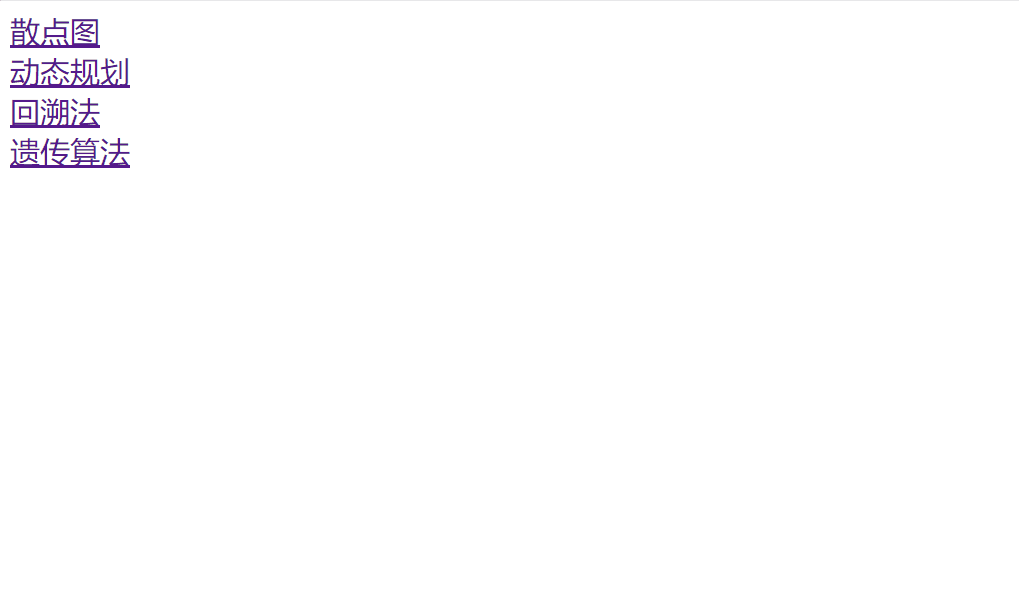（2）散点图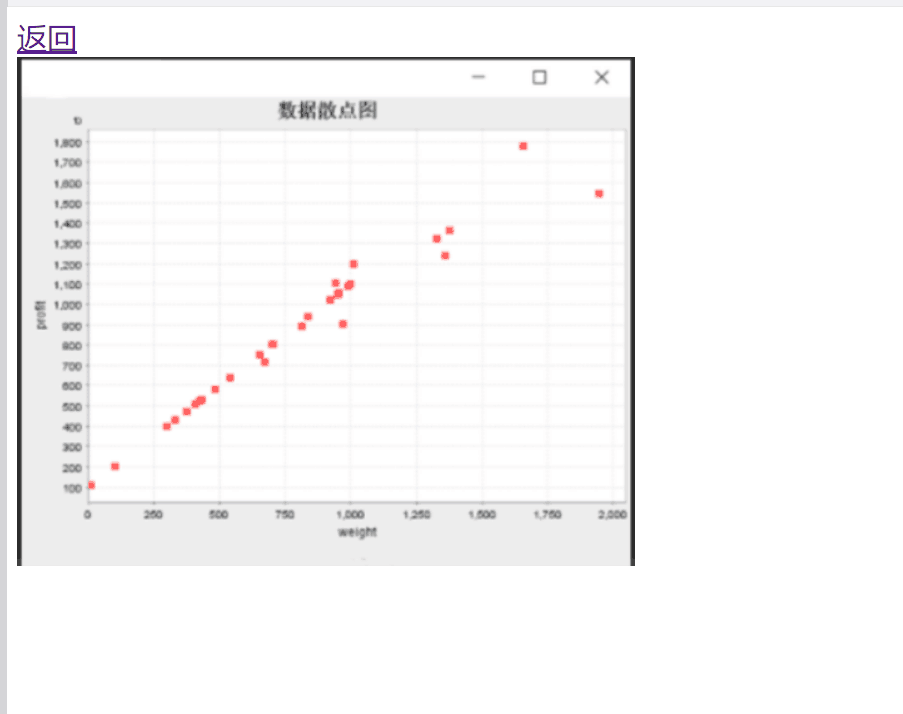（3）动态规划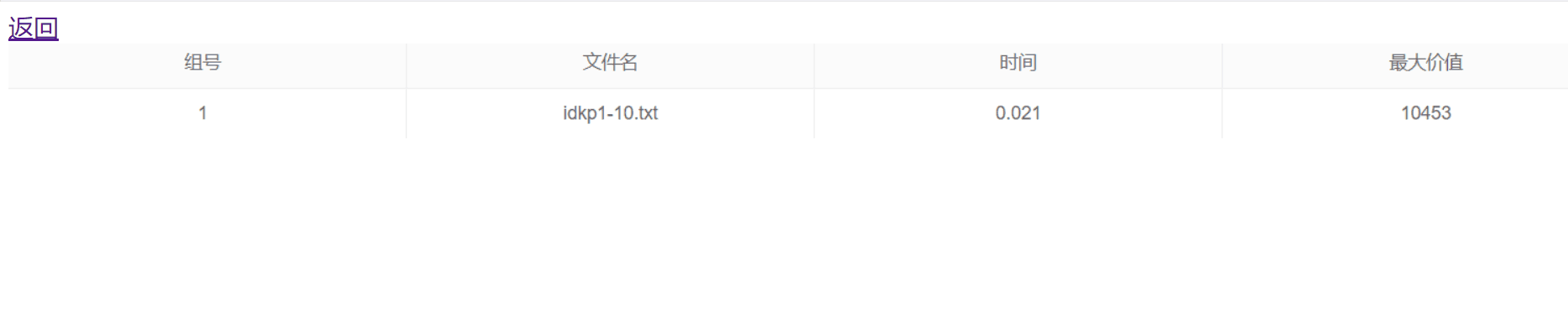（4）回溯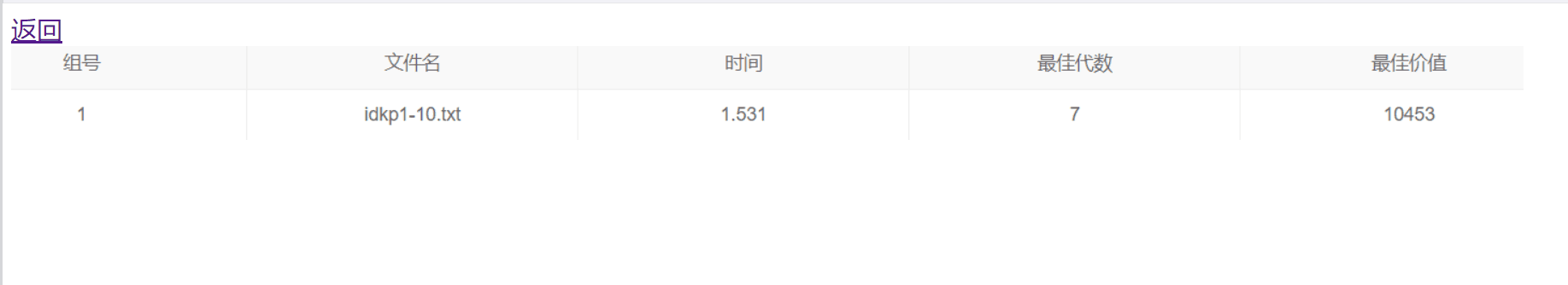（5）遗传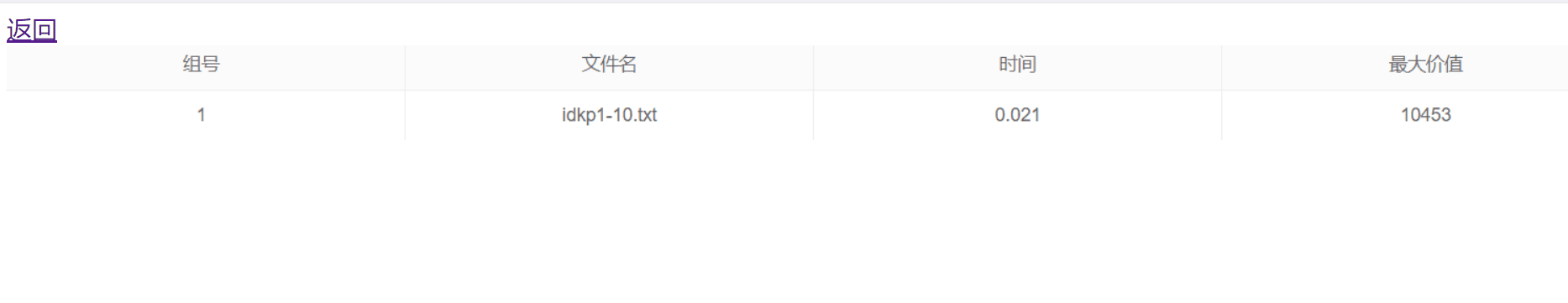##### 4.结对编程PSP2.1 任务内容 计划共完成需要的时间(min) 实际完成需要的时间(min)
Planning 计划 10 7
· Estimate · 估计这个任务需要多少时间，并规划大致工作步骤 8 5
Development 开发 820 970
·· Analysis 需求分析 (包括学习新技术) 20 20
· Design Spec · 生成设计文档 30 30
· Design Review · 设计复审 (和同事审核设计文档) 20 20
· Coding Standard 代码规范 (为目前的开发制定合适的规范) 20 20
· Design 具体设计 150 150
· Coding 具体编码 420 520
· Code Review · 代码复审 120 150
· Test · 测试（自我测试，修改代码，提交修改） 60 60
Reporting 报告 50 60
·· Test Report · 测试报告 20 30
· Size Measurement 计算工作量 10 10
· Postmortem & Process Improvement Plan · 事后总结 ,并提出过程改进计划 20 20

#### 小结感受：两人合作真的能够带来1+1>2的效果吗？通过这次结对合作，请谈谈你的感受和体会。

posted @ 2021-04-14 09:44  南通TheShy  阅读(84)  评论(0编辑  收藏  举报